# Metric tensor

(diff) ← Older revision | Latest revision (diff) | Newer revision → (diff)

basic tensor, fundamental tensor

A twice covariant symmetric tensor field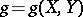on an-dimensional differentiable manifold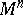,. The assignment of a metric tensor onintroduces a scalar product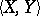of contravariant vectorson the tangent space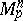ofat, defined as the bilinear function, where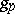is the value of the fieldat the point. In coordinate notation: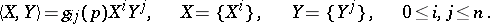The metric inwith this scalar product is regarded as infinitesimal for the metric of the manifold, which is expressed by the choice of the quadratic differential form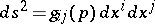(*)

as the square of the differential of the arc length of curves in, going fromin the direction. With respect to its geometric meaning the form (*) is called the metric form or first fundamental form on, corresponding to the metric tensor. Conversely, if a symmetric quadratic form (*) onis given, then there is a twice covariant tensor field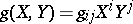associated with it and whose corresponding metric form is. Thus, the specification of a metric tensoronis equivalent to the specification of a metric form onwith a quadratic line element of the form (*). The metric tensor completely determines the intrinsic geometry of.

The collection of metric tensors, and the metric forms defined by them, is divided into two classes, the degenerate metrics, when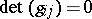, and the non-degenerate metrics, when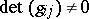. A manifoldwith a degenerate metric form (*) is called isotropic. Among the non-degenerate metric tensors, in their turn, are distinguished the Riemannian metric tensors, for which the quadratic form (*) is positive definite, and the pseudo-Riemannian metric tensors, when (*) has variable sign. A Riemannian (pseudo-Riemannian) metric introduced onvia a Riemannian (pseudo-Riemannian) metric tensor defines ona Riemannian (respectively, pseudo-Riemannian) geometry.

Usually a metric tensor, without special indication, means a Riemannian metric tensor; but if one wishes to stress that the discussion is about Riemannian and not about pseudo-Riemannian metric tensors, then one speaks of a proper Riemannian metric tensor. A proper Riemannian metric tensor can be introduced on any paracompact differentiable manifold.

How to Cite This Entry:
Metric tensor. Encyclopedia of Mathematics. URL: http://encyclopediaofmath.org/index.php?title=Metric_tensor&oldid=16631
This article was adapted from an original article by I.Kh. Sabitov (originator), which appeared in Encyclopedia of Mathematics - ISBN 1402006098. See original article